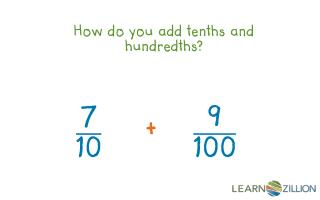# How do you add tenths and hundredths? - PowerPoint PPT PresentationDownload PresentationHow do you add tenths and hundredths?

How do you add tenths and hundredths?
Download Presentation## How do you add tenths and hundredths?

- - - - - - - - - - - - - - - - - - - - - - - - - - - E N D - - - - - - - - - - - - - - - - - - - - - - - - - - -
##### Presentation Transcript

1. In this lesson you will learn how to add tenths and hundredths by creating equivalent fractions.

2. Numerator: How many parts you have Denominator: How many equal parts make up a whole

3. When we add fractions with common denominators, that means the denominators are the same. = • +

4. + = + =

5. + = ?

6. + = ? x= + =

7. + = x= + =

8. In this lesson you learned how to add fractions with tenths and hundredths as denominators by creating equivalent fractions.

9. + = ?

10. Describe one extension activity here…

11. + = ? + = ?